GCSE Maths Number FDP Decimals

Multiplying And Dividing Decimals

# Multiplying And Dividing Decimals

Here we will learn about multiplying and dividing with decimals, including calculations involving integers and decimals, or just decimals using formal written methods.

There are also multiplying and dividing decimals worksheets based on Edexcel, AQA and OCR exam questions, along with further guidance on where to go next if you’re still stuck.

## What is multiplying and dividing decimals?

Multiplying and dividing decimals is the skill of carrying out a calculation involving multiplication and division where one or more of the values is a decimal.

For this skill the ability to multiply and divide by powers of ten is essential.

### What is multiplying and dividing decimals?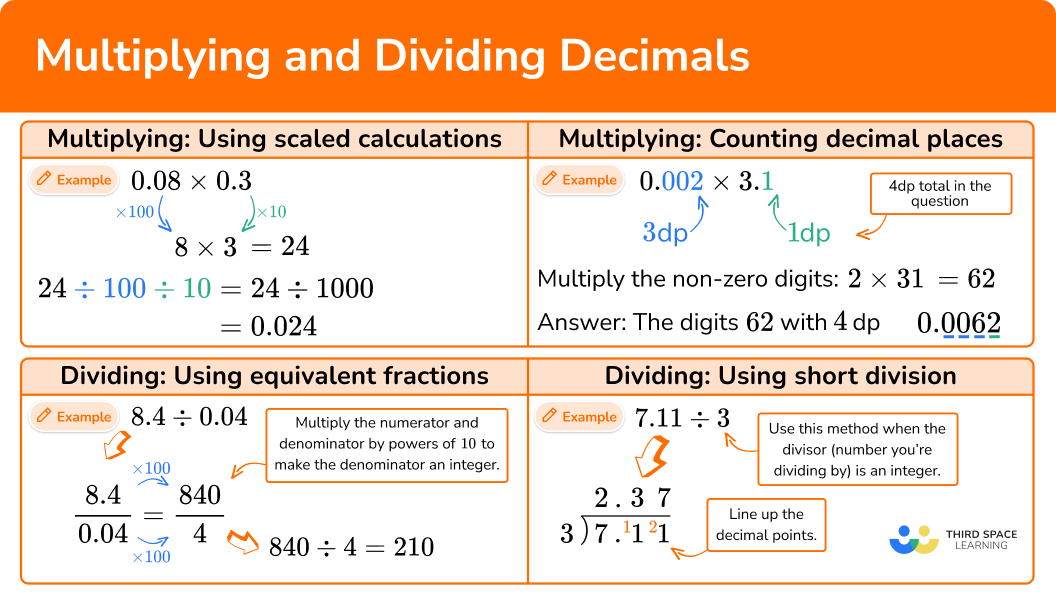### Multiplying with decimals

When multiplying with decimals we change any decimals in the calculation into integers (whole numbers) by multiplying by powers of 10. This gives us a scaled calculation which is easier to carry out. Remember when multiplying by 10 the decimal point doesn’t move, rather the digits move up changing their place value.

For example,

0.3 \times 0.07

3 \times 7 = 21

We now need to scale this answer down to find the solution to the original calculation. We do this by dividing by the same powers of 10 which we multiplied by in the first step. Remember when dividing by 10 the decimal point doesn’t move, rather the digits move down changing their place value.

21 \, {\color{orange} \div \, 10 \div 100} = 0.021

Another method of decimal multiplication, or as a way of checking your answer, is to count the number of decimal places. The combined number of decimal places in the question should be the same as the number of decimal places in the answer.

0.3 \; (1dp)\times0.07 \; (2dp)=0.021 \; (3dp)

### Dividing with decimals

• When dividing a decimal number by an integer we can use the standard short division method.

For example, 9.24 \div 6.

Sometimes trailing zeros may need to be written when the solution has more decimal places than the decimal number being divided.

For example,

0.9 \div 8.

• When dividing a number by a decimal we can use equivalent fractions to find an equivalent calculation which divides by an integer.

For example, 3 \div 0.2.

First we write this division as a fraction \cfrac{3}{0.2}.

Then we decide what to multiply 0.2 by in order to make it an integer. Most often we will multiply by a power of ten. We then write the equivalent fraction which has a denominator that is an integer.

Step-by-step guide: Equivalent fractions

This tells us that 3 \div 0.2 is equivalent to 30 \div 2 and therefore the answers to each of these calculations will be the same.

30 \div 2 = 15

Therefore 3 \div 0.2 = 15.

Sometimes a combination of equivalent fractions and short division is needed.

For example,

0.408 \div 0.03.

Multiplying and dividing decimals is used in many real life contexts.

For example,

• Money – Share £15.40 equally between two people. (£7.70)
• Speed / Distance / Time – It takes a vehicle 0.25 hours to travel 10.4 miles.
What is the average speed of the vehicle in miles per hour? (41.6{~mph})
• Mass – 8 slices of shortbread is made using 0.25{~kg} of brown sugar. How much brown sugar is needed for 10 slices? (0.3125{~kg})
• Area – A rectangular field measures 0.35{~km} by 0.41{~km}. What is the area of the field? (0.1435{~km^2})
• Converting units – Convert 6521 millilitres into litres. (6.521l)

## How to multiply with decimals

In order to multiply with decimals:

1. Change any decimals in the calculation into integers (whole numbers) by multiplying by powers of \bf{10} .
2. Calculate the answer to this scaled calculation.
3. Divide the answer to step 2 by the same powers of ten which you multiplied by in step 1.
4. Check your answer by ensuring that the combined number of decimal places in the question is the same as the number of decimal places in the answer.

### How to multiply with decimals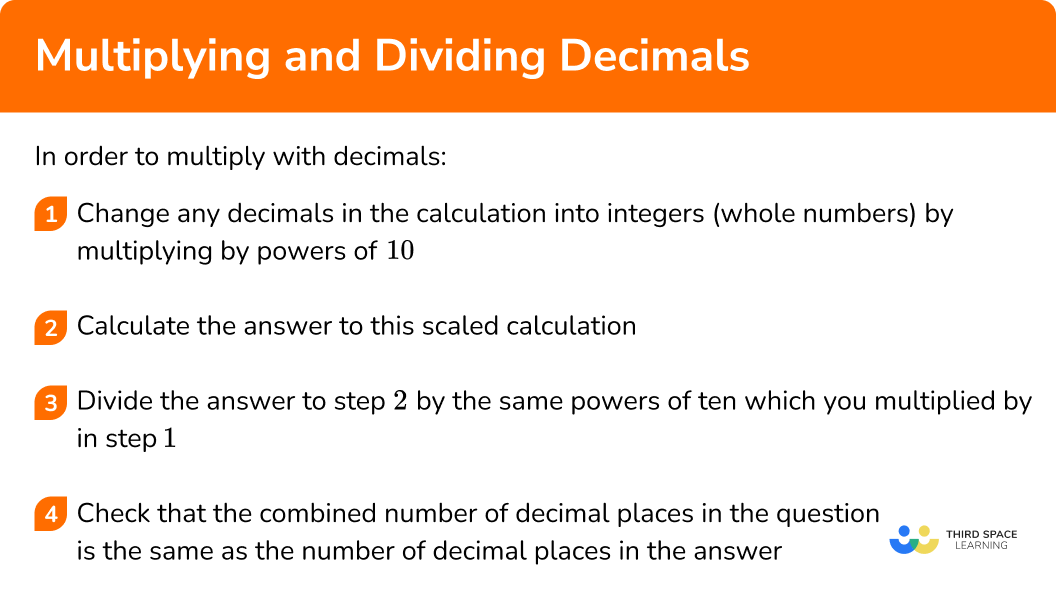### Related lessons on decimals

Multiplying and dividing decimals is part of our series of lessons to support revision on decimals. You may find it helpful to start with the main decimals lesson for a summary of what to expect, or use the step by step guides below for further detail on individual topics. Other lessons in this series include:

## Multiplying decimals examples

### Example 1: multiplying an integer by a decimal

Calculate 9 \times {0.07}.

1. Change any decimals in the calculation into integers (whole numbers) by multiplying by powers of \bf{10}.

Multiply 0.07 by 100 to make the integer 7.

9 \times {0.07}

\color{orange} \times {100}

9 \times {7} (This is the scaled calculation.)

2Calculate the answer to this scaled calculation.

9 \times {7} = 63

3Divide the answer to step 2 by the same powers of ten which you multiplied by in step 1.

In step 1 we multiplied by 100 so now we divide by 100.

63 \, {\color{orange} \div \, {100}} = 0.63

4Check your answer by ensuring that the combined number of decimal places in the question is the same as the number of decimal places in the answer.

9 \times {0.07} \; (2dp) = 0.63 \; (2dp)

### Example 2: multiplying two decimals

Calculate 0.003 \times {0.2}.

Multiply 0.003 by 1000 to make the integer 3.

Multiply 0.2 by 10 to make the integer 2.

0.003 \times {0.2}

\color{orange} \times \, {1000} \times{10}

3 \times {2} (This is the scaled calculation.)

3 \times {2} = 6

In step 1 we multiplied by 1000 and by 10 so now we divide by 1000 and by 10.

6 \, {\color{orange} \div \, {1000} \, \div {10}} = 0.0006

0.003 \; (3dp) \times {0.2} \; (1dp) = 0.0006 \; (4dp)

### Example 3: multiplying with decimals when long multiplication is needed

Calculate 18 \times {3.5}.

Multiply 3.5 by 10 to make 35.

18 \times {3.5}

\quad \; \color{orange} \times \, {10}

18 \times {35} (This is the scaled calculation.)

Use a long multiplication method of your choice such as this column method.

18 \times {35} = 630

In step 1 we multiplied 3.5 by 10 so now we divide by 10.

630 \div {10} = 63.0

18 \times {3.5} \; (1dp) = 63.0 \; (1dp)

As 63.0 is the same as 63, the answer can be written in either format, unless the question specifies writing the answer to 1 decimal place.

Note that the last digit in the number 630 is a zero and so when we divided by 10, we still wrote this digit in its place value because this then matches the number of decimal places in the question.

## How to divide with decimals

In order to divide with decimals:

1. Check if the divisor is an integer or a decimal.
(a) Integer – go to step 3.
(b) Decimal – write the calculation as a fraction.
2. Write an equivalent fraction which has a denominator that is an integer.
Note: The best option is often to multiply the numerator and denominator by a power of ten. Then check if the fraction simplifies before moving onto step 3.
3. Use short division to calculate the answer.

### How to divide with decimals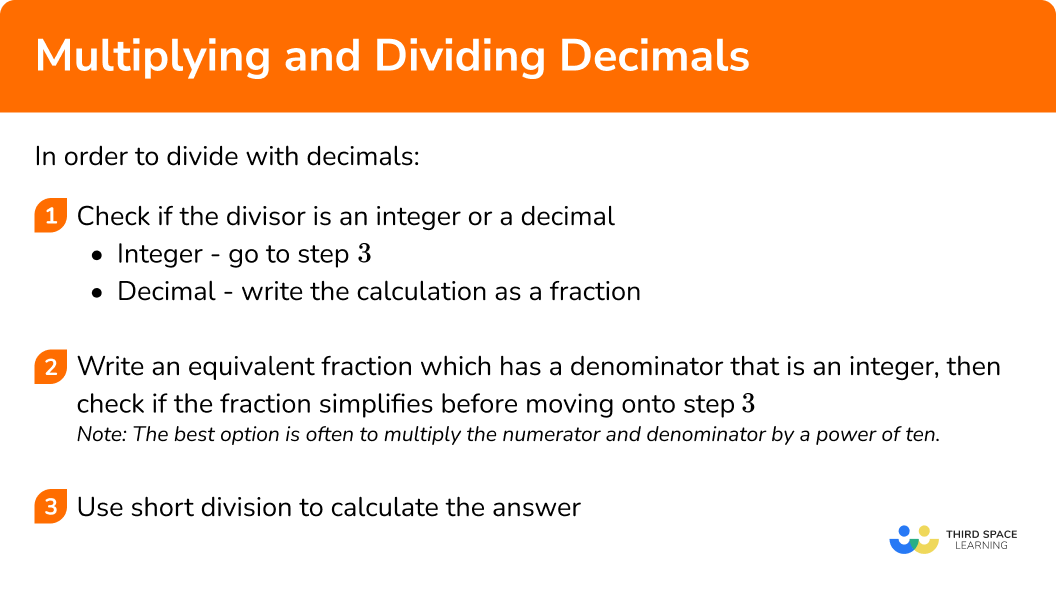## Dividing decimals examples

### Example 4: dividing a decimal by an integer

Calculate 5.34 \div {2}.

The divisor (the number we are dividing by) is an integer (2) so we can go to step 3.

### Example 5: dividing a decimal by an integer

Calculate 2.9 \div {8}.

The divisor (the number we are dividing by) is an integer (8) so we can go to step 3.

### Example 6: dividing a decimal by a decimal

Calculate 4.2 \div {0.6}.

The divisor (the number we are dividing by) is a decimal (0.6) so we write the calculation as a fraction.

\cfrac{4.2}{0.6}

Note: The best option is often to multiply the numerator and denominator by a power of ten. Then check if the fraction simplifies before moving onto step 3.

We could simplify the fraction here but you might also spot that 42 \div {6} = 7 .

As the numbers in this question are part of the times tables we can calculate the answer mentally without the need for short division.

42 \div {6} = 7

### Example 7: dividing a decimal by a decimal

Calculate 4.95 \div {2.5}.

The divisor (the number we are dividing by) is a decimal (2.5) so we write the calculation as a fraction.

\cfrac{4.95}{2.5}

Note: The best option is often to multiply the numerator and denominator by a power of ten. Then check if the fraction simplifies before moving onto step 3.

Note that we only need the denominator to be an integer.

We could now simplify the fraction by dividing by 5.

Calculate 49.5 \div {5}.

### Common misconceptions

• Getting mixed up between the ‘scaled calculations’ method when multiplying with decimals and ‘equivalent fractions’ method when dividing with decimals
When we multiply with decimals we can use a scaled calculation. This means the answer we get must be scaled back at the end to find the final answer. When we use equivalent fractions to help us divide with decimals, the fractions we write are equivalent (they have exactly the same value) and do not need adapting at the end.

Students can sometimes get these methods mixed up and forget to scale back when multiplying decimals, or attempt to adapt an answer that was already correct when dividing with decimals.

Remember: Multiplying with decimals uses scaled calculations and therefore the answer to this needs scaling back to find the final answer.
Dividing by a decimal uses equivalent fractions which are totally equal calculations and therefore the answer does not need changing.

• Not accounting for zero digits when multiplying with decimals
When multiplying with decimals we can check our answer by ensuring the combined number of decimal places in the question is equal to the number of decimal places in the answer. However, when zero digits are involved we have to be careful using this rule and remember to account for them.

0.7 \; (1dp) \times {0.8}\; (1dp) = 0.56(2dp)
Here we can see the rule works.

0.5 \; (1dp) \times {0.8} \; (1dp) = 0.4 \;(1dp)
Here it might appear that the rule doesn’t work.

However, because 5\times{8} = 40, the original answer would be 0.5 \; (1dp) \times {0.8} \; (1dp) = 0.40 \; (2dp) and then the trailing zero has been removed.

Watch out for integers that multiply to give a number that ends in zero when using the counting digits rule for checking your answer.

• Putting the numbers the wrong way round when using short division
Let’s describe a short division set up as a ‘bus stop’. One number goes inside the bus stop and the other goes outside the bus stop. The answer to the short division goes on top of the bus stop. Students can sometimes get the numbers the wrong way round, particularly when dividing with decimals.

Remember that the divisor (the number you are dividing by) goes on the outside of the bus stop, and the number you are dividing goes on the inside of the bus stop.
For example, 1.4\div{2} should be written like this,

Carrying out the division, we have

So 1.4\div{2}=0.7.

When writing a fraction as a short division the denominator goes on the outside of the bus stop and the numerator goes on the inside of the bus stop.

\cfrac{3.3}{8}

Carrying out the division, we have

### Practice multiplying and dividing decimals questions

1) Calculate 0.4 \times {8}.

3.20.320.488.40.4 \times {8}

\color{orange} \times \, {10}

4 \times {8} = 32

32 \, {\color{orange} \div \, {10}} = 3.2

2) Multiply together 0.03 and 0.05.

0.000150.150.0150.0015Multiply 0.03 by 100 to get 3.

Multiply 0.05 by 100 to get 5.

3 \times {5} = 15

100 \times {100} = 10000

Dividing 15 by 10000, we have

15\div{10000}=0.0015

This means that 0.03\times{0.05}=0.0015

3) Calculate 1.2 \times {0.05}.

0.060.66.00.0061.2 \times {0.05}

\color{orange} \times \, {10} \, \times {100}

12\times {5} = 60

60 \, {\color{orange} \div \, {10} \, \div {100}} = 0.060 = 0.06

4) Divide 12.4 by 8.

1.541.5515.415.5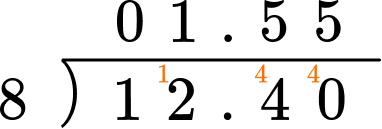5) Calculate the value of 1.435 \div {0.05}.

287028.70.2870.00287Write the division as a fraction \cfrac{1.435}{0.05}.

Write an equivalent fraction which has a denominator that is an integer.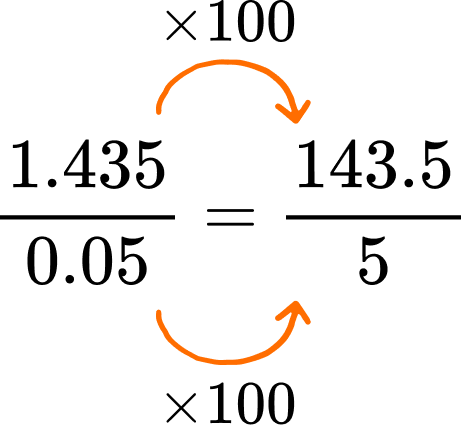Now using short division,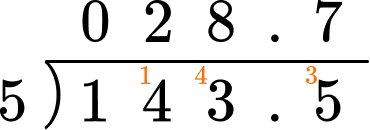6) Calculate the value of 84 \div {0.6}.

141404201.4Write the division as a fraction \cfrac{84}{0.6}.

Write an equivalent fraction which has a denominator that is an integer.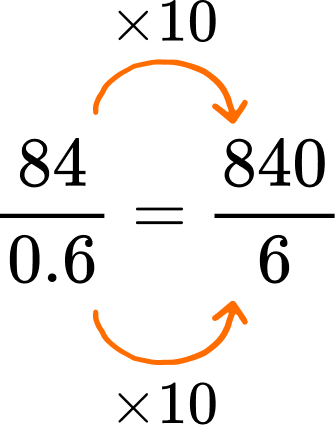We could now simplify the fraction.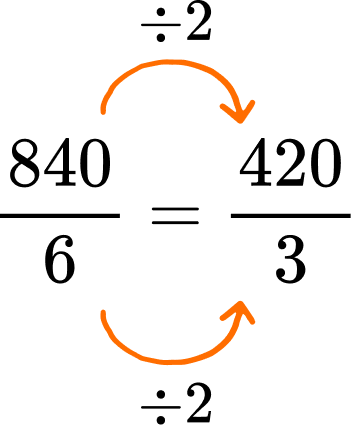Lastly using short division,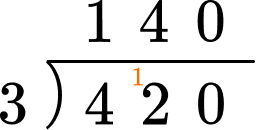### Multiplying and dividing decimals GCSE questions

1. Calculate

(a) 4.5 \div {9}

(b) 3.5 \div {0.07}

(3 marks)

(a) 4.5 \div {9} = 0.5

(1)

(b) 3.5 \div {0.07} = \cfrac{3.5}{0.07} = \cfrac{350}{7}

(1)

50

(1)

2. The piece of ribbon is 7.8 metres long. The ribbon is going to be cut into 5 pieces of equal length. How long will each piece of ribbon be?

(2 marks)

7.8 \div {5}

(1)

= 1.56

(1)

3. A sandpit is 1.2 metres long and 0.7 metres wide. Calculate the area of the sandpit giving your answer in metres squared.

(2 marks)

1.2 \times {0.7}

(1)

=0.84

(1)

4. Two positive numbers less than 1 are multiplied together to give 0.08. Give one possible pair of values.(1 mark)

Either of the following pairs of numbers (in any order),

0.2(0) \times {0.4(0)}

(1)

0.1(0) \times {0.8(0)}

(1)

## Learning checklist

You have now learned how to:

• Carry out multiplication and division in calculations involving decimals using written methods to show your working out

## Still stuck?

Prepare your KS4 students for maths GCSEs success with Third Space Learning. Weekly online one to one GCSE maths revision lessons delivered by expert maths tutors.

Find out more about our GCSE maths tuition programme.